A Source Book in Greek Science

Contents:
Author: Euclid

Show Summary
Geometry

# POSTULATES

Euclid, Elements I, Postulates. Translation of T. L. Heath, The Thirteen Books of Euclid’s Elements I. 154

Let the following be postulated:1

1. To draw a straight line from any point to any point.

2. To produce a finite straight line continuously in a straight line.

3. To describe a circle with any centre and distance.

4. That all right angles are equal to one another.

5. That, if a straight line falling on two straight lines make the interior angles on the same side less than two right angles, the two straight lines, if produced indefinitely, meet on that side on which are the angles less than the two right angles.

# The Parallel Postulate

Proclus Diadochus, Commentary on Euclid’s Elements I, pp. 191.21–193.9 (Friedlein). Translation of T. L. Heath, The Thirteen Books of Euclid’s Elements I. 202–2032

This [fifth postulate] ought even to be struck out of the Postulates altogether; for it is a theorem involving many difficulties which Ptolemy, in a certain book, set himself to solve, and it requires for the demonstration of it a number of definitions as well as theorems. And the converse of it is actually proved by Euclid himself as a theorem. It may be that some would be deceived and would think it proper to place even the assumption in question among the postulates as affording, in the lessening of the two right angles, ground for such an instantaneous belief that the straight lines converge and meet. To such as these Geminus correctly replied that we have learned from the very pioneers of this science not to have any regard to mere plausible imaginings when it is a question of the reasonings to be included in our geometrical doctrine. For Aristotle says that it is as justifiable to ask scientific proofs of a rhetorician as to accept mere plausibilities from a geometer; and Simmias is made by Plato to say that he recognizes as quacks those who fashion for themselves proofs from probabilities. So in this case the fact that, when the right angles are lessened, the straight lines converge is true and necessary; but the statement that, since they converge more and more as they are produced, they will sometime meet is plausible but not necessary, in the absence of some argument showing that this is true in the case of straight lines. For the fact that some lines exist which approach indefinitely, but yet remain non-secant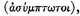although it seems improbable and paradoxical, is nevertheless true and fully ascertained with regard to other species of lines.1 May not then the same thing be possible in the case of straight lines which happens in the case of the lines referred to? Indeed, until the statement in the Postulate is clinched by proof, the facts shown in the case of other lines may direct our imagination the opposite way. And, though the controversial arguments against the meeting of the straight lines should contain much that is surprising, is there not all the more reason why we should expel from our body of doctrine this merely plausible and unreasoned (hypothesis)?

It is then clear from this that we must seek a proof of the present theorem, and that it is alien to the special character of postulates. But how it should be proved, and by what sort of arguments the objections taken to it should be removed, we must explain at the point where the writer of the Elements is actually about to recall it and use it as obvious. It will be necessary at that stage to show that its obvious character does not appear independently of proof, but is turned by proof into matter of knowledge.

# THE METHOD OF EXHAUSTION

Euclid, Elements VII, Proposition 2. Translation of T. L. Heath, The Thirteen Books of Euclid’s Elements III. 371–373

Circles are to one another as the squares on the diameters.

Let ABCD, EFGH be circles, and BD, FH their diameters; I say that, as the circle ABCD is to the circle EFGH, so is the square on BD to the square on FH.

For, if the square on BD is not to the square on FH as the circle ABCD is to the circle EFGH,

then, as the square on BD is to the square on FH, so wilt the circle ABCD be either to some less area than the circle EFGH, or to a greater.

First, let it be in that ratio to a less area S.

Let the square EFGH be inscribed in the circle EFGH;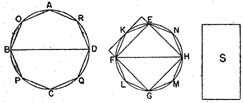then the inscribed square is greater than the half of the circle EFGH, inasmuch as, if through the points E, F, G, H we draw tangents to the circle, the square EFGH is half the square circumscribed about the circle, and the circle is less than the circumscribed square;

hence the inscribed square EFGH is greater than the half of the circle EFGH.

Let the circumferences EF, FG, GH, HE be bisected at the points K, L, M, N,

and let EK, KF, FL, LG, GM, MH, HN, NE be joined;

therefore each of the triangles EKF, FLG, GMH, HNE is also greater than the half of the segment of the circle about it, inasmuch as, if through the points K, L, M, N we draw tangents to the circle and complete the parallelograms on the straight lines EF, FG, GH, HE, each of the triangles EKF, FLG, GMH, HNE will be half of the parallelogram about it,

while the segment about it is less than the parallelogram;

hence each of the triangles EKF, FLG, GMH, HNE is greater than the half of the segment of the circle about it.1

Thus, by bisecting the remaining circumferences and joining straight lines, and by doing this continually, we shall leave some segments of the circle which wilt be less than the excess by which the circle EFGH exceeds the area S.

For it was proved by the first theorem of the tenth book that, if two unequal magnitudes be set out, and if from the greater there be subtracted a magnitude greater than the half, and from that which is left a greater than the half, and if this be done continually, there will be left some magnitude which will be less than the lesser magnitude set out.

1 Let segments be left such as described, and let the segments of the circle EFGH on EK, KF, FL, LG, GM, MH, HN, NE be less than the excess by which the circle EFGH exceeds the area S.

Therefore the remainder, the polygon EKFLGMHN, is greater than the area S.

Let there be inscribed, also, in the circle ABCD the polygon AOBPCQDR similar to the polygon EKFLGMHN; therefore, as the square on BD is to the square on FH, so is the polygon AOBPCQDR to the polygon EKFLGMHN.     [XII. 1]2

But, as the square on BD is to the square on FH, so also is the circle ABC to the area S;

therefore also, as the circle ABCD is to the area S, so is the polygon AOBPCQDR to the polygon EKFLGMHN;     [V. 11]3

therefore, alternately, as the circle ABCD is to the polygon inscribed in it, so is the area S to the polygon EKFLGMHN.     [V. 16]4

But the circle ABCD is greater than the polygon inscribed in it; therefore the area S is also greater than the polygon EKFLGMHN.

But it is also less:

which is impossible.

Therefore, as the square on BD is to the square on FH, so is not the circle ABCD to any area less than the circle EFGH.

Similarly we can prove that neither is the circle EFGH to any area less than the circle ABCD as the square on FH is to the square on BD.

I say next that neither is the circle ABCD to any area greater than the circle EFGH as the square on BD is to the square on FH.

For, if possible, let it be in that ratio to a greater area S.

Therefore, inversely, as the square on FH is to the square on DB, so is the area S to the circle ABCD.

But, as the area S is to the circle ABCD, so is the circle EFGH to some area less than the circle ABCD;

therefore also, as the square on FH is to the square on BD, so is the circle EFGH to some area less than the circle ABCD:     [V. 11]

which was proved impossible.

Therefore, as the square on BD is to the square on FH, so is not the circle ABCD to any area greater than the circle EFGH.

And it was proved that neither is it in that ratio to any area less than the circle EFGH;

therefore, as the square on BD is to the square on FH, so is the circle ABCD to the circle EFGH

Therefore, etc.1     Q.E.D.

# THE "PYTHAGOREAN" THEOREM

Euclid, Elements I, Proposition 47. Translation of T. L. Heath, The Thirteen Books of Euclid’s Elements I. 349–350

In right-angled triangles the square on the side subtending the right angle is equal to the squares on the sides containing the right angle.

Let ABC be a right-angled triangle having the angle BAC right;

I say that the square on BC is equal to the squares on BA, AC.

For let there be described on BC the square BDEC, and on BA, AC the squares GB, HC;     [I. 46]

through A let AL be drawn parallel to either BD or CE, and let AD, FC be joined.

Then, since each of the angles BAC, BAG is right, it follows that with a straight line BA, and at the point A on it, the two straight lines AC, AG not lying on the same side make the adjacent angles equal to two right angles;

therefore CA is in a straight line with AG.

[I. 14]

For the same reason

BA is also in a straight line with AH.

And, since the angle DBC is equal to the angle FBA:

for each is right:

let the angle ABC be added to each;

therefore the whole angle DBA is equal to the whole angle FBC.     [C.N. 2]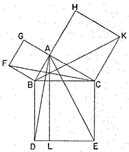And, since DB is equal to BC, and FB to BA, the two sides AB, BD are equal to the two sides FB,BC respectively;

and the angle ABD is equal to the angle FBC;

therefore the base AD is equal to the base FC,

and the triangle ABD is equal to the triangle FBC.     [I. 4]

Now the parallelogram BL is double of the triangle ABD, for they have the same base BD and are in the same parallels BD, AL.     [I. 41]

And the square GB is double of the triangle FBC,

for they again have the same base FB and are in the same parallels FB, GC.

[I. 41]

But the doubles of equals are equal to one another.

Therefore the parallelogram BL is also equal to the square GB.

Similarly, if AE, BK be joined, the parallelogram CL can also be proved equal to the square HC;

therefore the whole square BDEC is equal to the two squares GB,HC.

[C.N. 2]

And the square BDEC is described on BC, and the squares GB,HC on BA, AC.

Therefore the square on the side BC is equal to the squares on the sides BA, AC.

Therefore etc.1     Q.E.D.

# THE GOLDEN SECTION

Euclid Elements II, Proposition 11. Translation of T. L. Heath, The Thirteen Books Euclid’s Elements I. 402

To cut a given straight line so that the rectangle contained by the whole and one of the segments is equal to the square on the remaining segment.1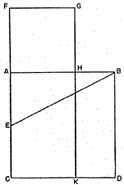Let AB be the given straight line;

thus it is required to cut AB so that the rectangle contained by the whole and one of the segments is equal to the square on the remaining segment.

For let the square ABDC be described on AB;     [I. 46]

let AC be bisected at the point E, and let BE be joined;

let CA be drawn through to F, and let EF be made equal to BE;

let the square FH be described on AF, and let GH be drawn through to K.

I say that AB has been cut at H so as to make the rectangle contained by AB, BH equal to the square on AH.

For, since the straight line AC has been bisected at E, and FA is added to it,

the rectangle contained by CF, FA together with the square on AE is equal to the square on EF.     [II. 6]

But EF is equal to EB;

therefore the rectangle CF, FA together with the square on AE is equal to the square on EB.

But the squares on BA, AE are equal to the square on EB, for the angle at A is right;

therefore the rectangle CF, FA together with the square on AE is equal to the squares on BA, AE.

Let the square on AE be subtracted from each;

therefore the rectangle CF, FA which remains is equal to the square on AB.

Now the rectangle CF, FA is FK, for AF is equal to FG;

and the square on AB is AD;

therefore FK is equal to AD.

Let AK be subtracted from each;

therefore FH which remains is equal to HD.

And HD is the rectangle AB, BH, for AB is equal to BD;

and FH is the square on AH;

therefore the rectangle contained by AB, BH is equal to the square on HA;

therefore the given straight line AB has been cut at H so as to make the rectangle contained by AB, BH equal to the square on HA.     Q.E.F.

# THE HORN-ANGLE

Euclid, Elements III, Proposition 16. Translation of T. L. Heath, The Thirteen Books of Euclid’s Elements II. 37–39

The straight line drawn at right angles to the diameter of a circle from its extremity will fall outside the circle, and into the space between the straight line and the circumference another straight line cannot be interposed; further the angle of the semicircle is greater, and the remaining angle less, than any acute rectilineal angle.

Let ABC be a circle about D as centre and AB as diameter;

I say that the straight line drawn from A at right angles to AB from its extremity will fall outside the circle.

For suppose it does not, but, if possible, let it fall within as CA, and let DC be joined.

Since DA is equal to DC,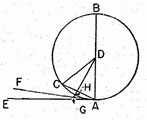the angle DAC is also equal to the angle ACD.     [I. 5]

But the angle DAC is right;

therefore the angle ACD is also right:

thus, in the triangle ACD, the two angles DAC, ACD are equal to two right angles: which is impossible.     [I. 17]

Therefore the straight line drawn from the point A at right angles to BA will not fall within the circle.

Similarly we can prove that neither will it fall on the circumference;

therefore it will fall outside.

Let it fall as AE;

I say next that into the space between the straight line AE and the circumference CHA another straight line cannot be interposed.

For, if possible, let another straight line be so interposed, as FA, and let DG be drawn from the point D perpendicular to FA.

Then, since the angle AGD is right,

and the angle DAG is less than a right angle,

AD is greater than DG.     [I. 19]

But DA is equal to DH;

therefore DH is greater than DG, the less than the greater: which is impossible,

Therefore another straight line cannot be interposed into the space between the straight line and the circumference.

I say further that the angle of the semicircle contained by the straight line BA and the circumference CHA is greater than any acute rectilineal angle,

and the remaining angle contained by the circumference CHA and the straight line AE is less than any acute rectilineal angle.

For, if there is any rectilineal angle greater than the angle contained by the straight line BA and the circumference CHA, and any rectilineal angle less than the angle contained by the circumference CHA and the straight line AE, then into the space between the circumference and the straight line AE a straight line will be interposed such as will make an angle contained by straight lines which is greater than the angle contained by the straight line BA and the circumference CHA, and another angle contained by straight lines which is less than the angle contained by the circumference CHA and the straight line AE.

But such a straight line cannot be interposed;

therefore there will not be any acute angle contained by straight lines which is greater than the angle contained by the straight line BA and the circumference CHA, nor yet any acute angle contained by straight lines which is less than the angle contained by the circumference CHA and the straight line AE.1

PORISM. From this it is manifest that the straight line drawn at right angles to the diameter of a circle from its extremity touches the circle.

Q.E.D.

1 The postulates, in connection with the definitions and axioms, constitute the principles of Euclidean geometry. The first three are postulates of construction. The fourth is required in order that the fifth may be applied. The fifth, which is probably Euclid’s own attempt to found a sound theory of parallels, has given rise to the greatest controversy. Not only were unsuccessful efforts made to prove the postulate, but non-Euclidean geometries were developed in modern times on the basis of a denial of the postulate. [Edd.]

1 The reference is to curves like the hyperbola and conchoid, which have asymptotes. [Edd.]

1 In order to show that by doubling the number of sides of the inscribed polygon indefinitely, the area of the circle will be "exhausted," Euclid seeks to show that the difference in area between any polygon in the series and the circle is less than half the difference between the preceding polygon and the circle. [Edd.]

1 From this point on the proof is given in modern notation by Heath as follows: "Now let X, X′ be the areas of the circles, d, d′ their diameters, respectively.

Then, if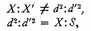where S is some area either greater or less than X′.

I. Suppose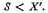Continue the construction of polygons in X′ until we arrive at one which reaves over segments together less than the excess of X′ over S, i.e. a polygon such that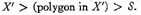Inscribe in the circle X a polygon similar to that in X′.

Then (polygon in X):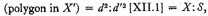by hypothesis;

and, alternately,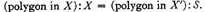But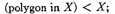therefore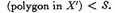But, by construction,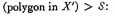which is impossible.

Hence S cannot be less than X′ as supposed.

II. Suppose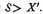Since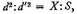we have, inversely,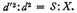Suppose that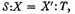whence, since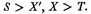[V. 14]

Consequently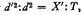where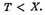This can be proved impossible in exactly the same way as shown in Part I.

Hence S cannot be greater than X′ as supposed.

Since then S is neither greater nor less than X′,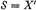, and therefore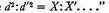2 Similar polygons inscribed in circles are to one another as the squares on the diameters. [Edd.]

3 This proposition states, in effect, that if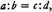and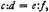then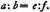[Edd.]

4 This proposition states, in effect, that if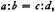then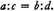[Edd.]

1 This form of the proof may be due to Eudoxus (see p. 36). Note that Archimedes, in applying the method of exhaustion (e.g., in Measurement of a Circle, Prop. I, p. 60, below), proved the second part by circumscribing successive polygons. It was thus unnecessary for him to reduce the second Case to the first. Eudemus attributed a proof of this proposition to Hippocrates of Chios (see p. 55). The nature of his proof is not known. [Edd.]

1 This is the famous "windmill" proof of the so-called "Pythagorean theorem." The tradition connecting Pythagoras with this theorem is not clear, and it may be doubted that he had arrived at a general proof. In any case, the proof as given by Euclid is probably an original proof for the purpose of including the theorem in Book I rather than postponing it to the books on proportion.

Whether or not the Pythagoreans had a general proof, their work in the theory of numbers indicates an acceptance of its truth (see pp. 21–23). [Edd.]

1 This is essentially the problem of dividing a line into extreme and mean ratio: the smaller segment is to the larger as the larger is to the whole line. The construction given is held by Heath to be pre-Euclidean; an alternative method is given later by Euclid (VI.30).

The solution of the problem is necessary in order to construct the regular pentagon, and hence the regular dodecahedron, one of the five so-called "Platonic figures."

The ratio of the larger segment to the whole line is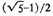(approximately .618). A rectangle of unit length with height equal to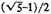was thought to have the most beautiful proportions. Because of such aesthetic considerations the names "golden section" and "golden rectangle" came into use in modern times. [Edd.]

1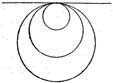The nature of the angle between the diameter and the circumference and of the so-called" horn-angle," the angle between the tangent and the circumference or between two tangent circumferences, was the subject of widespread discussion in antiquity. The angle between the diameter and the circumference seems to decrease, while the angle between the tangent and the circumference seems to increase as the circles become smaller. And yet the former angle always remains greater, and the latter angle smaller than any acute rectilineal angle.

This paradox may well have exercised men like Zeno of Elea, Protagoras, Democritus, and Eudoxus. Not only were concepts of tangency and of angle involved, but, like so many other paradoxes of the Greeks, the nature of continuity itself.

The controversy continued in the Middle Ages and in modern times. Among those who dealt with the problem were Campanus in the thirteenth century, Cardan, Peletier, and Vieta in the sixteenth, and Galileo and Wallis in the seventeenth For a summary of recent studies on the horn angle (including that formed by non-circular arcs) see E. Kasner, "The Recent Theory of the Horn Angle," Scripta Mathematica 11 (1945) 263–267. [Edd.]

Contents:

### Related Resources

Euclid
Life of Euclid

Title: A Source Book in Greek Science

Select an option:

## Email Options

Title: A Source Book in Greek Science

Select an option: